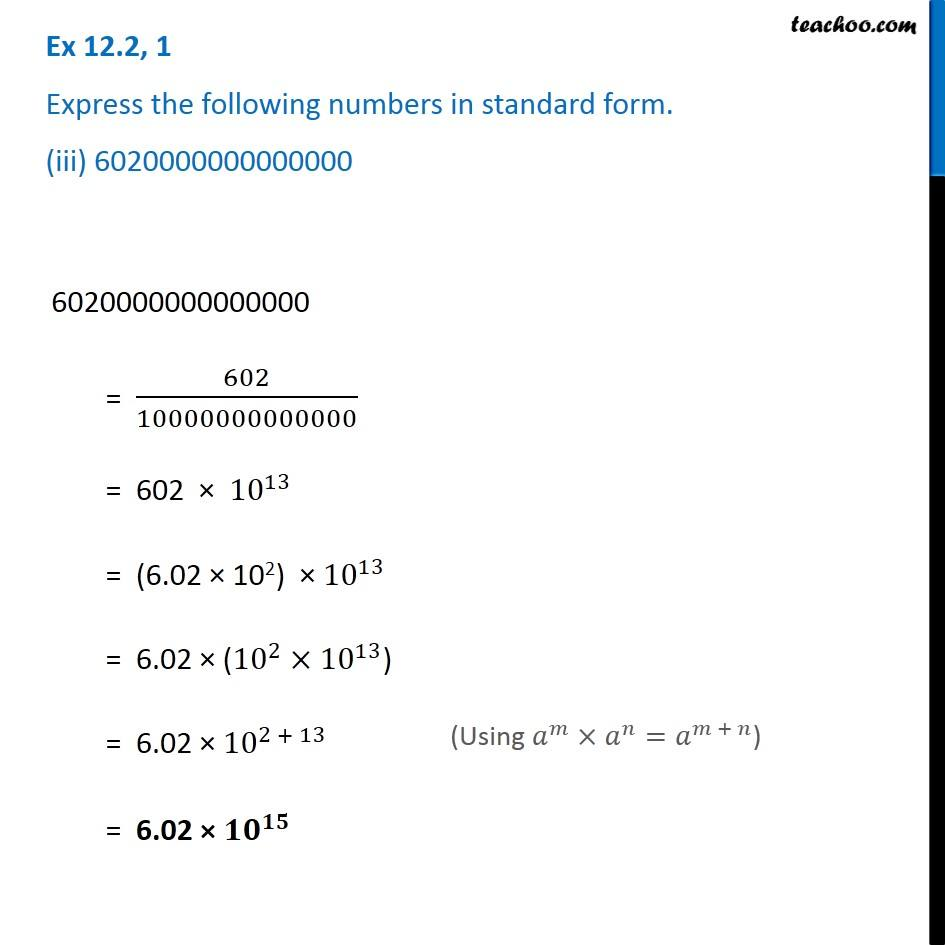Ex 12.2

Chapter 12 Class 8 Exponents and Powers
Serial order wiseGet live Maths 1-on-1 Classs - Class 6 to 12

### Transcript

Ex 12.2, 1 Express the following numbers in standard form. (iii) 6020000000000000 6020000000000000 = 602/10000000000000 = 602 × 〖10〗^13 = (6.02 × 102) × 〖10〗^13 = 6.02 × (〖10〗^2×〖10〗^13) = 6.02 × 〖10〗^(2 + 13) = 6.02 × 〖𝟏𝟎〗^𝟏𝟓 (Using 𝑎^𝑚×𝑎^𝑛=𝑎^(𝑚 + 𝑛))